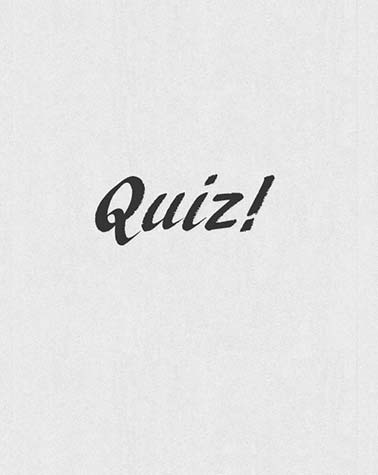# Unit 3 Review Practice

13 Questions | Total Attempts: 143Settings• 1.
We use this unit to measure force
• A.

Newtons

• B.

Mass

• C.

Grams

• D.

MPH

• 2.
If a book is resting on a table and the forces are equal and opposite. The forces are ...
• A.

Unbalanced

• B.

Balanced

• C.

Option 3

• D.

Option 4

• 3.
"For every action, there is an equal and opposite reaction" This is which Law?
• A.

Newton's First Law

• B.

Newton's Second Law

• C.

Newton's Third Law

• D.

Option 4

• 4.
If you are on a roller coaster and it stops suddenly, how does inertia affect the riders?
• A.

The riders still move forward until stopped by a harness

• B.

The riders stop exactly when the ride stops

• C.

The riders move backwards

• D.

Option 4

• 5.
A force that resists movement between two surfaces is called...
• A.

Inertia

• B.

Kinetic

• C.

Potential

• D.

Friction

• 6.
The amount of matter in an object is called...
• A.

Mass

• B.

Inertia

• C.

Velocity

• D.

Option 4

• 7.
The distance covered during a period of time
• A.

Acceleration

• B.

Speed

• C.

Velocity

• D.

Option 4

• 8.
What is velocity?
• A.

Speed and direction

• B.

Speed and inertia

• C.

Speed and force

• D.

Option 4

• 9.
Seat belts in a car prevent people from riders getting injured during a crash, Which nlaw do the belts help with?
• A.

Newton's First Law

• B.

Newton's Second Law

• C.

Newton's Third Law

• D.

Option 4

• 10.
This type of motion moves in an arc (or parabola)
• A.

Straight line

• B.

Projectile

• C.

Vibrational

• D.

Circular

• 11.
A spinning top moves in this type of motion...
• A.

Straight line

• B.

Projectile

• C.

Circular

• D.

Vibrational

• 12.
What are the unseen forces that make objects fall back down after they are thrown in the air?
• A.

Gravity and friction

• B.

Gravity and inertia

• C.

Inertia and Acceleration

• D.

Option 4

• 13.
Which things affect the amount of force an object has?
• A.

Shape

• B.

Mass

• C.

Ingredients

• D.

AccelerationBack to top